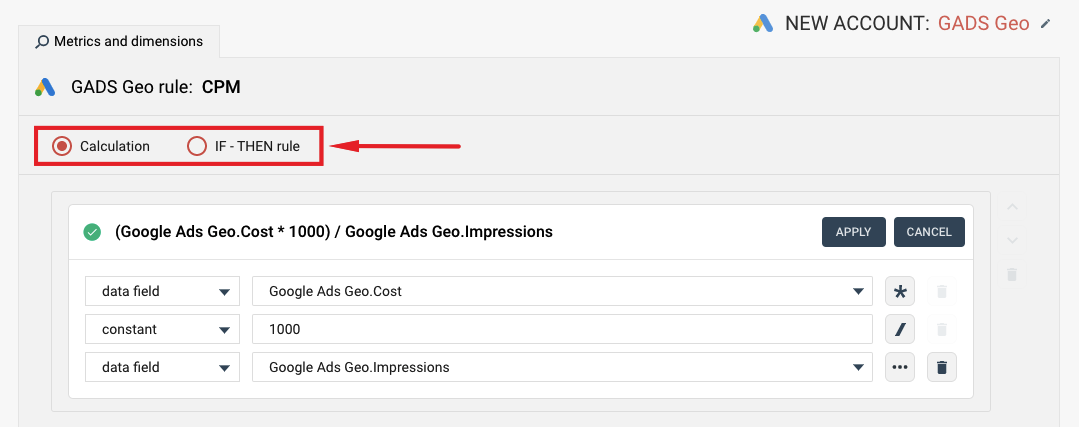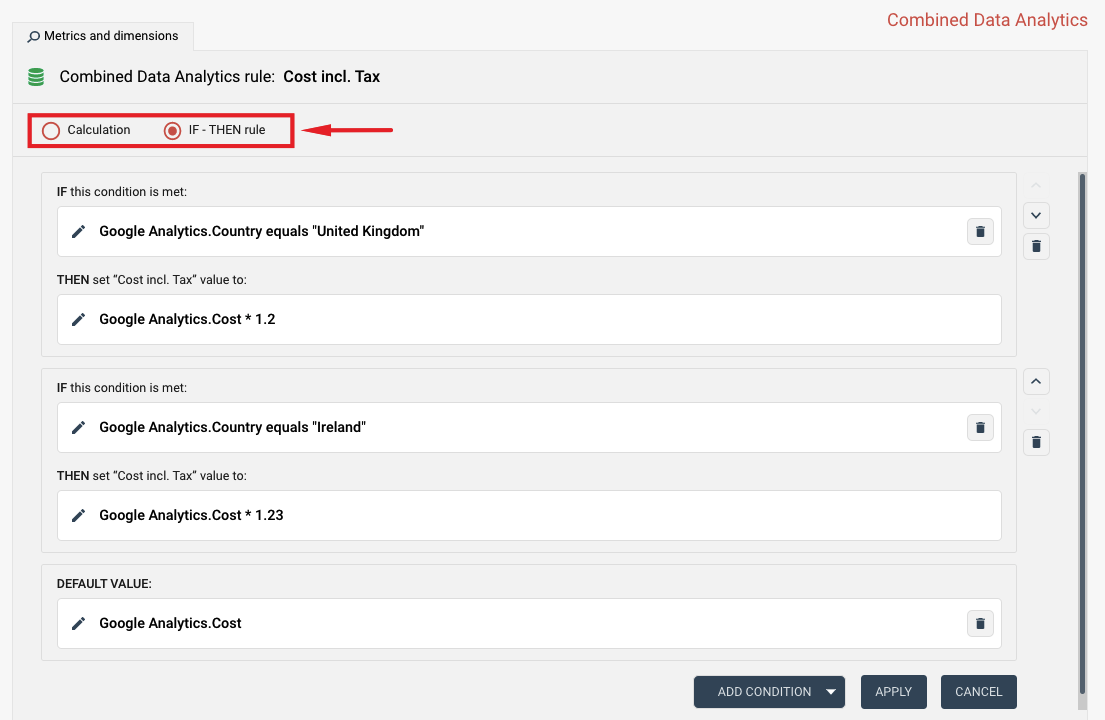EnglishTRIALTRIALEnglish

# CALCULATIONS AND IF-THEN CONDITIONS IN RULES

Knowledge BaseCalculations And If-then Conditions In Rules

## Calculations and IF-THEN conditions in rules

When you create a new rule, you can select either Calculation or IF-THEN rule.

• Calculation is a formula-based rule that uses numeric functions or text manipulations. It has no IF conditions. Calculations are used to build metrics such as CostPerClick = Cost / Clicks.
• IF-THEN rule uses multiple IF conditions. It allows building complex cases such as applying different VAT rates depending on the country.

Below is an example of the Calculation rule (CostPerMille = Cost / Impressions * 1000):More complex Conditional logic can be build using IF-THEN rules. Here is an example of a rule that calculates VAT based on the Country field value (add 23% VAT to all sales in Ireland and 20% to sales in the UK).For more details on how to create such a metric and use the IF-THEN rule, please read this tutorial.

You can also check some of our advanced rule examples in the following tutorials:SUGGESTED TUTORIALS